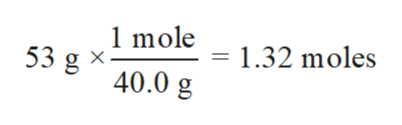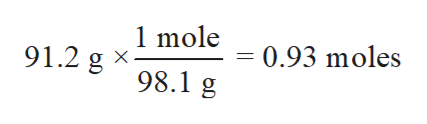Aqueous sulfuric acidH2SO4will react with solid sodium hydroxideNaOHto produce aqueous sodium sulfateNa2SO4and liquid waterH2O.Suppose 91.2 g of sulfuric acid is mixed with 53. g of sodium hydroxide. Calculate the minimum mass of sulfuric acid that could be left over by the chemical reaction. Be sure your answer has the correct number of significant digits.

Question

Aqueous sulfuric acid

H2SO4

will react with solid sodium hydroxide

NaOH

to produce aqueous sodium sulfate

Na2SO4

and liquid water

H2O

.Suppose 91.2 g of sulfuric acid is mixed with 53. g of sodium hydroxide. Calculate the minimum mass of sulfuric acid that could be left over by the chemical reaction. Be sure your answer has the correct number of significant digits.

Step 1

In chemistry, there are many ways to check the deviation of experimental data from the accurate value. These different methods provide the difference between accuracy and precisions of the experimental data which can be further used to calculate the error percentage in the experiment.

Step 2

Calculate moles of sodium hydroxide:

Molar mass of NaOH= 40.0 g/mol

Mass of NaOH = 53 ghelp_outlineImage Transcriptionclose1 mole 1.32 moles 53 g = 40.0 g fullscreen
Step 3

Calculate moles of sulfuric acid:

Molar mass of sulfuric acid...help_outlineImage Transcriptionclose1 mole = 0.93 moles 91.2 g 98.1 g fullscreen

Want to see the full answer?

See Solution

Want to see this answer and more?

Our solutions are written by experts, many with advanced degrees, and available 24/7

See Solution
Tagged in

Chemistry